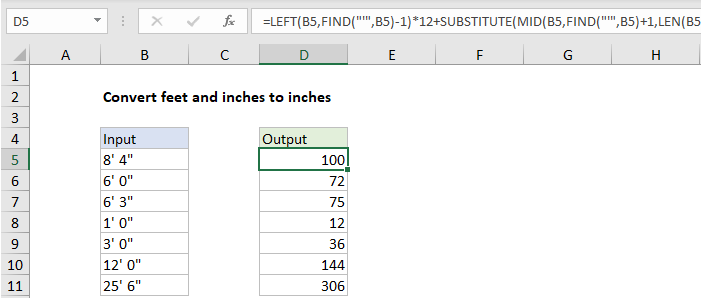## Excel Office

Excel How Tos, Tutorials, Tips & Tricks, Shortcuts

# Convert feet and inches to inches in Excel

To convert a measurement in feet and inches to inches only (i.e. 4’5″ to 53) you can use a formula based on several functions, including LEFT, FIND, MID, and SUBSTITUTE. See illustration below:

## Formula

```=LEFT(B5,FIND("'",B5)-1)*12+SUBSTITUTE
(MID(B5,FIND("'",B5)+1,LEN(B5)),"""","")```

##Explanation

In the example shown, the formula in D5 is:

```=LEFT(B5,FIND("'",B5)-1)*12+SUBSTITUTE
(MID(B5,FIND("'",B5)+1,LEN(B5)),"""","")```

### How this formula works

In the first part of the formula, feet are extracted and converted LEFT and FIND like this:

`=LEFT(B5,FIND("'",B5)-1)*12`

Working from the inside out, FIND is used to locate the position of the single quote (‘) in the string. This number (minus one) goes into the LEFT function as the number of characters to extract. For cell B5, LEFT returns 8, which is then multiplied by 12 to get inches.

Worked Example:   Manipulating text strings using Left, Mid, Right, Len, Substitute in Excel

Note: LEFT will return text, but multiplying by 12 will covert the text a number.

In the second part of the formula, we extract the inches part of the text value with this:

`SUBSTITUTE(MID(B5,FIND("'",B5)+1,LEN(B5)),"""","")`

Here we again locate the position of the single quote (‘) in the string with FIND. This time however we add one, and feed the result into the MID function as the starting point for extracting characters.

Worked Example:   Highlight rows that contain in Excel

For the number of characters to extract, we cheat and use the LEN function. LEN will return the total characters in B5, which is a larger number than exist after the single quote in the string. however, MID will simply extract all remaining characters. For B5, MID will return ” 4″””, which goes into the SUBSTITUTE function as text.

Worked Example:   Join cells with comma in Excel

SUBSTITUTE is configured to look for a double quote (“”””) and replace with nothing (“”). In the case of B5, SUBSTITUTE returns ” 4″ as text, which is added to the feet number already determined. As before, the math operation converts the text a number, and Excel returns a final result of 100.

### Other units

Once you have the measurement in inches, you can use the CONVERT function to convert to other units of measure.